Loading 2020 NBA Most Improved Player of the Year Futures...
2020 NBA Most Improved Player of the Year Odds
-155
0.65 to 1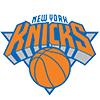Julius Randle
43.2% implied probability

+140
1.4 to 1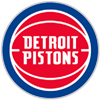Jerami Grant
29.6% implied probability

+1400
14 to 1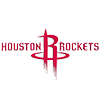Christian Wood
4.7% implied probability

+3000
30 to 1Nikola Jokic
2.3% implied probability

+3000
30 to 1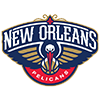Zion Williamson
2.3% implied probability

+3500
35 to 1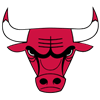Zach LaVine
2.0% implied probability

+5000
50 to 1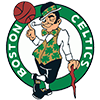Jaylen Brown
1.4% implied probability

+5000
50 to 1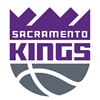De'Aaron Fox
1.4% implied probability

+8000
80 to 1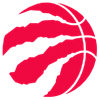Chris Boucher
0.9% implied probability

+10000
100 to 1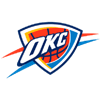Luguentz Dort
0.7% implied probability

+10000
100 to 1Collin Sexton
0.7% implied probability

+10000
100 to 1Shai Gilgeous-Alexander
0.7% implied probability

+10000
100 to 1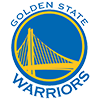Andrew Wiggins
0.7% implied probability

+10000
100 to 1Domantas Sabonis
0.7% implied probability

+10000
100 to 1Lonzo Ball
0.7% implied probability

+15000
150 to 1Jarrett Allen
0.5% implied probability

+15000
150 to 1OG Anunoby
0.5% implied probability

+15000
150 to 1RJ Barrett
0.5% implied probability

+15000
150 to 1Donte DiVincenzo
0.5% implied probability

+15000
150 to 1Michael Porter
0.5% implied probability

+15000
150 to 1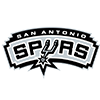Keldon Johnson
0.5% implied probability

+15000
150 to 1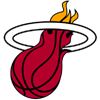Tyler Herro
0.5% implied probability

+15000
150 to 1Dejounte Murray
0.5% implied probability

+15000
150 to 1Mikal Bridges
0.5% implied probability

+15000
150 to 1Ja Morant
0.5% implied probability

+15000
150 to 1Darius Bazley
0.5% implied probability

+15000
150 to 1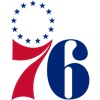Shake Milton
0.5% implied probability

+15000
150 to 1Jayson Tatum
0.5% implied probability

+25000
250 to 1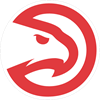Kevin Huerter
0.3% implied probability

+25000
250 to 1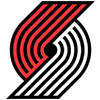Norman Powell
0.3% implied probability

+25000
250 to 1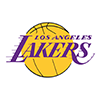Talen Horton-Tucker
0.3% implied probability

+25000
250 to 1Deandre Ayton
0.3% implied probability

+25000
250 to 1Kyle Kuzma
0.3% implied probability

+25000
250 to 1Devin Booker
0.3% implied probability

+25000
250 to 1Jaxson Hayes
0.3% implied probability